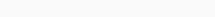# Math Puzzles Puzzle 71 Answer AndroidMath puzzle game level 71 solution with detailed hints and explanation, Scroll below to find out.

Math puzzles increase your logical thinking. The objective of the game is to find patterns hidden in the puzzle in order to arrive at the solution. Challenge yourself with different levels of math puzzles and stretch the limits of your intelligence.

### Math Puzzles Puzzle 71:

if 1,3,1=69

2,1,3=187

3,2,4=276

4,3,7=363

then 5,1,9=?Solution/Hint:

100-31=69

200-13=187

300-24=276

400-37=363

500-19=481

If there is any Doubt or you are unable to understand the solution, then please let me know in comments, I will surely help you.

### One thought on “Math Puzzles Game Level 71 Answer with Solution”

•February 3, 2020 at 05:44